# How to Sum Data Every N Rows in Excel

Sometimes in statistic, data is grouped by every N rows, for example if data is grouped by week, then data is grouped every 7 rows (or columns in some cases). In our daily life, we may meet many cases like this. It is necessary for us to have the knowledge of sum data by every N rows in excel. Actually, excel built-in functions SUM and OFFSET can help us resolve this issue properly.

This article will show you ‘sum every N rows’ based on SUM and OFFSET functions. We will introduce the two functions with simple examples, descriptions, screenshots and explanations, and we will let you know the formula works process with these functions. Actually, the key point in sum every n rows is the usage of OFFSET function, so in this article, we focus on introduce OFFSET function syntax, arguments and how it works with SUM function, we also provide simple example to illustrate the function. After reading the following article, I’m sure you can have a simple understanding of OFFSET function, besides, you can learn well on sum data every N rows. You can work well with these functions in your daily work in the future.

EXAMPLE: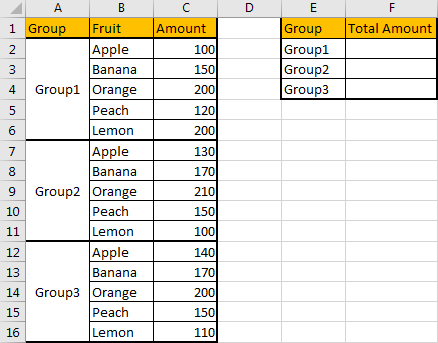There are three groups of fruit combination, we want to sum total amount for each group. We can see that each group has 5 kinds of fruits, so in fact, start from C2 (apple amount in group1), we need to sum data every 5 rows. So, here’s our question, how can we sum data every 5 rows? And how to apply the same formula in cell F2/F3/F4 to sum total amount correctly (enter a formula in F2, then we can copy the formula to F3 and F4 to sum data directly).

FORMULA: SUM & OFFSET FUNCTIONS

Step 1: In cell F2, enter the formula =SUM(OFFSET(\$C\$2,(ROW()-2)*5,0,5,1)).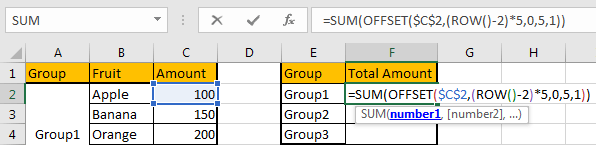Step 2: Click Enter to get return value. Verify that 770 is calculated correctly. 100+150+200+120+200=770, the formula works properly.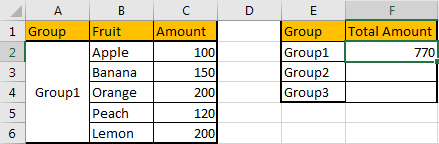Step 3: Copy the formula to F3 and F4. Directly drag down the fill handle to fill F3 and F4.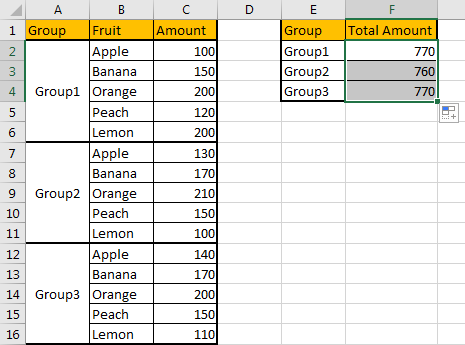Check the return value. All values are calculated correctly. This formula is applied properly for F3 and F4, that means the formula works well on ‘sum every 5 rows’.

HOW THIS FORMULA WORKS:

This formula contains two excel functions SUM and OFFSET. SUM function is commonly used in daily work, but OFFSET function is not as widely used as SUM function, so we will explain the formula started from this function.

Simply, we can think that OFFSET function can create a dynamic range based on the given starting point, number of rows under the starting point, and other reference.

Syntax: =OFFSET (reference, rows, cols, [height], [width])

We can see that it has five arguments, the last two arguments are optional. We will explain these five arguments with simply description.

Reference: starting point, it can be a cell or a range;

Rows: N rows under the starting row;

Cols: N columns offset to the right of the starting point;

Height: height in rows of the returned range;

Width: width in columns of the returned range.

For example, see the table below.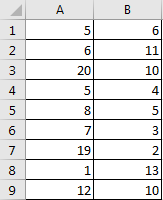In any cell, enter the formula =OFFSET(A2,1,0). In this formula, the starting point is A2, 1 (rows) means only one row below A2, so we come to A3; 0 (cols) means 0 column offsets to A2; above all, we get A3 value ‘20’ finally by applying the formula.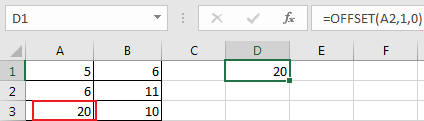We can also get an array by using OFFSET function.

Still in this instance, in D2 enter the formula =OFFSET(A2,1,1,5,1).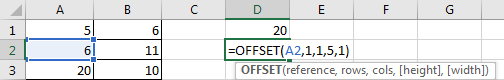Press ‘Ctrl+Shift+Enter’, verify that formula is enclosed in brackets {}.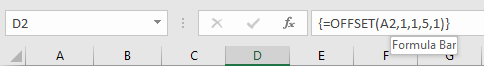In formula bar, press F9, you can get an array {10;4;5;3;2} as the returned result. Compare with table, we can know the array reflects the range B3:B7.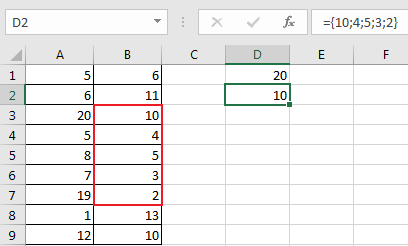For formula =OFFSET(A2,1,1,5,1), refer to former instance, we can know that we come to B3 if only applying =OFFSET(A2,1,1). The formula returns a range with 5 rows and 1 column by the last two optional arguments (5,1).

Now, refer to above example and explanation, I think you are simply known the OFFSET function now. So, we will start to explain the whole formula in our instance from inside to outside.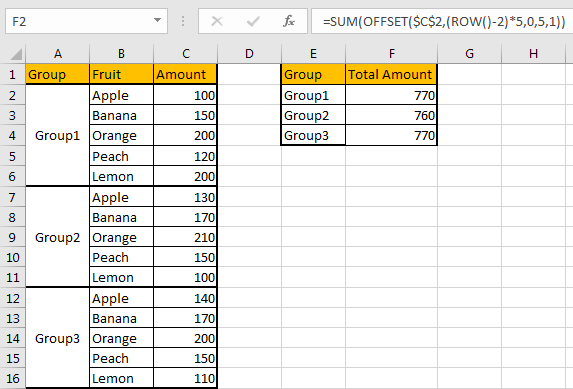See above screenshot, in cell F2 we enter the formula =SUM(OFFSET(\$C\$2,(ROW()-2)*5,0,5,1)). In this formula, for OFFSET part:

Reference: the starting point. Obviously, we want to sum data from range C2:C6 into F2, so we enter \$C\$2 here. As we also want to apply the formula in F3/F4, so we lock this cell (add \$) to keep it also as the start point for the formula in F3 and F4.

Rows: In this case, it is a formula (ROW()-2)*5.

First, ROW() returns current cell row number. So, for F2 the row number is 2, we get 2 by function ROW(); when applying the formula to F3, we will get 3, and for F4, we will get 4.

For cell F2, the sum range is C2:C6, the starting point C2 is included in this range, so for Rows argument, we want to enter 0; the formula (ROW()-2)*5 equals to (ROW(F2)-2)*5=0, just satisfy our demands.

For cell F3, the sum range is C7:C11, 5 rows below the starting point C2, so we want to enter 5 for Rows argument; the formula (ROW()-2)*5 equals to (ROW(F3)-2)*5=5.

For cell F4, the sum range is C12:C16, 10 rows below the starting point C2, so we want to enter 10 for Rows argument; the formula (ROW()-2)*5 equals to (ROW(F4)-2)*5=10. Now, we can say that (ROW()-2)*5 can provide correct number of rows below the starting point.

Cols: As no column offsets to the starting point, so enter 0 here.

Height: As the returned result is C2:C6, 5 rows 1 column, so we enter 5 here.

Width: Similar to Height argument, we enter 1.

Now let’s see how this formula works step by step:

Step 1: Enter the formula into F2.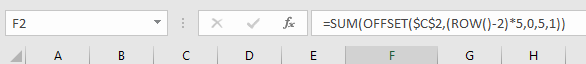Step 2: ROW()-2 returns 0 in formula bar.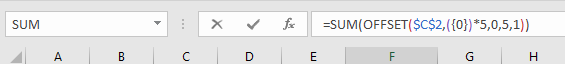Step 3: 0*5=5, so the formula converts to =SUM(OFFSET(\$C\$2,{0},0,5,1)).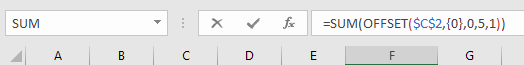Step 4: In SUM(OFFSET(\$C\$2,{0},0,5,1)), OFFSET(\$C\$2,{0},0,5,1) returns an array {100;150;200;120;200}.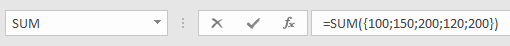Step 5: SUM function can sum data in the array.

For SUM function, the syntax is:

=SUM(number1,[number2],…)

So we get 770 finally.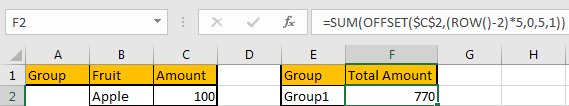RESULT:

Let’s check formula work process for F3.

Step 1: Enter the same formula into F3. This time ROW() returns 1.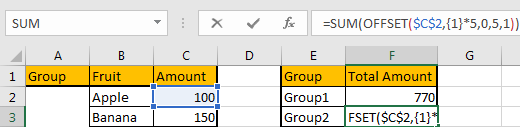Step 2: Formula updates to =SUM(OFFSET(\$C\$2,{5},0,5,1)).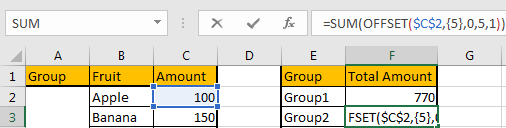Step 3: OFFSET(\$C\$2,{5},0,5,1) returns array {130;170;210;150;100}.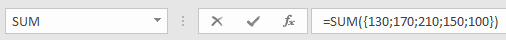Step 4: The final result is 130+170+210+150+100=760.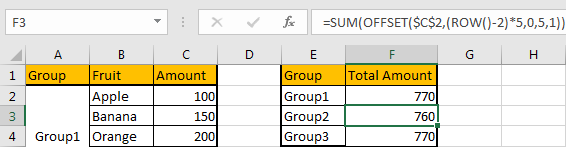You can get the same process for F4.

NOTE:

For OFFSET function, the key point is to determine the starting point. If it is fixed, we need to add \$ before row and column to lock it.

### Related Functions

• Excel SUM function
The Excel SUM function will adds all numbers in a range of cells and returns the sum of these values. You can add individual values, cell references or ranges in excel.The syntax of the SUM function is as below:= SUM(number1,[number2],…)…